# Measures

MEASURES (LENGTH)

• Length is the distance between two points.
• The basic units for measuring length is metres.
• The standard units for length are Kilometres and metres.

Units of length from the biggest to the smallest.

Km    Hm    Dm    m      dm    cm     mm

1        0        0         0          0          0          0

1        0         0          0           0        0

1          0           0          0        0

1            0        0        0

1        0        0

1        0

Expressing metres as centimetres

1. Change 6m to cm.

m      dm    cm

1        0        0

1m = 100cm

Big to small you multiply  (x)

6m     =        (6 x 100)

=        600cm

1. Express 2 ½ m as cm

m      dm    cm

1        0        0

1m     =        100cm

Big to small =        (x)

2 ½ m         =        2 + ½

=        (2 x 100)cm +(1/2 x 100)cm

=        200cm         +        50cm

=        250cm.

Or;

Change 2 ½ m into an improper fraction

2 1/2m       =        5/2m

=        5/2 x 100

=        5 x 50

=        250cm

WEEK  SEVEN.

LESSON  TWO.

Expressing centimetres to metres

1. Change 400m to metres

m  dm    cm

1   0        0

100cm    =        1m

mall to big you divide  (÷)

400cm             = 400

100

=        4 ÷ 1

=        4m

1. Change 1900cm to metres

m  dm    cm

1   0        0

100cm    =        1m

Small to big you divide (÷)

1900cm           = 1900

100

=        19 ÷ 1

=        19m

WEEK  SEVEN.

LESSON  THREE.

Converting centimetres to metres and cm

1. Convert 120cm to metres and cm

m  dm    cm

1      0        0

100cm    =        1m

120cm    =        100cm + 20cm

Small to big you divide       (÷)

120cm             = 100 +        20cm

100

=        1 ÷ 1  +        20cm

=        1m 20cm

1. Change 840cm to metres and cm.

m  dm    cm

1   0        0

100cm    =        1m

840cm    =        800cm + 40cm

Small to big     =        (÷)

840cm             = 800 +        20cm

100

=        8 ÷ 1  +        40cm

=        8m 40cm

LESSON  FOUR.

1. Add: 2m 45cm           +        6m  36cm

1

m       cm                        SW       45

2        45                                + 36

6        36  81

8       81

1

m       cm                        SW       25 110

8        25                               + 85 100

6        85   110 1 r 10

15      10

LESSON  FIVE.

Subtraction of metres and centimetres

1. Subtract:         m       cm SW

6        80                           80

– 2        60 – 60

4  20  20

1. M       cm SW

9        24                         1m = 100ccm                 0 x 124

5        30 100 + 24 = 124 –      30

3        94   094

1. Tom had 3m 70cm of cloth. He used 1m 20cm. How much cloth remained?

m       cm SW

3        70                           70

– 1        20 – 20

2  50  50

LESSON  ONE.

Multiplication of metres and centimetres

1. Multiply:  6m   25cm x 6

m       cm                        SW       25 150

61 25                               x  6 100

x          6   150 1 r 50

37      50

1. Mary, Ben and Kareen bought a cloth 3m  45cm each. What was the total size of the cloth?

m       cm                        SW       45 135

31 45                               x  3 100

x          3   135 1 r 35

10      53

LESSON  TWO.

Expressing Km to metres

1. Change 7Km to metres

Km         Hm    Dm    m

1               0        0        0

1Km       =        1000m

7Km       =        (7 x 1000)m

=        7000m

1. Change 4 ½ Km o metres

Km         Hm    Dm    m

1            0        0        0

1Km       =        1000m

4 ½ Km  =        4 + ½ Km

=        (4 x 1000)m +        (1/2 x 1000)m

=        4000m        +        500m

=        4500m

LESSON  THREE.

Expressing metres to Km.

1. Change 3000m as Km.

1000m   =        1Km

3000m   = 3000 Km

1000

=        3 ÷ 1

=        3Km

1. Express 2000m as Km.

1000m   =        1Km

2000m   = 2000 Km

1000

=        2 ÷ 1

= 2Km

LESSON  FOUR.

Perimeter of a Rectangle and Square

Find the perimeter of the following.Perimeter = Length + Width + Length + Width

=        L + W = L = W

=        (12cm + 4cm) + (12cm + 4cm)

=        16cm + 16cm

=        32cmPerimeter    = Side + Side +Side +Side  =            S + S + S + S

=        6cm + 6cm +6cm + 6cm

=        36cm

WEEK  EIGHT.

LESSON  Five.

Area of a rectangle and a square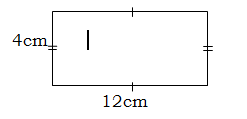Area    = L X W

= 12 cm x 4 cm

=    48 cm2.Area =       S X S

=   6 cm x 6 cm

= 36 cm2

WEEK  NINE.

LESSON  ONE.

Area and Perimeter of a triangleArea of a rectangle     =        L X W

=        6cm x 4cm

24cm2

_________________________________________________________________________Area of the shaded part is half the area of the rectangle.

=        24cm2 ÷ 2

= 12cm2

Area of a triangle            =        ½ x base x height

=        ½ x b x h

=        ½ x 6cm x 4cm

= 12cm2

Find the area of the triangle.
Height         =        3cm

Base            =        4cm                   height = 3cm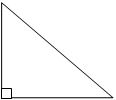Area            =        ½ x b x h                       Base = 4cm

=        ½ x 4cm x 3cm

=        2cm x 3cm

=        6cm2

_________________________________________________________________________Calculate the Perimeter

Perimeter    =        S + S + S

=        10cm + 4cm + 3cm

= 17cm

Find the area and perimeter of the triangle shown.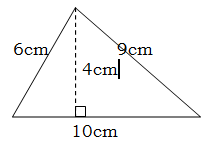Base            =        10cm

Height         =        4cm

Area            =        ½ x b x h

=        ½ x 10cm x 4cm

=        10cm x 2cm

= 20cm2

________________________________________________________________________Perimeter    =        S + S + S

=        9cm + 6cm + 10cm

=        15cm + 10cm

=        25cm

WEEK  NINE.

LESSON  TWO.

Finding the missing side of a rectangle

1. The area of a rectangle is 24cm2. If its length is 6cm, find its width.

Sketch: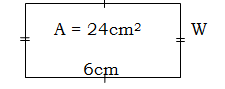L        x        W      =        A

6cm   x        W       =        24cm2

6Wcm=        24cm2

W       = 24 cm2

6cm

W       =        4cm

_________________________________________________________________________Find the length of a rectangle whose width is 5cm if its area is 60cm2.

Sketch: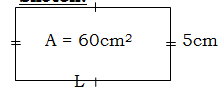L        x        W      =        A

L        x        5cm   =        60cm2

5Lcm=         60cm2

L        = 60 cm2

5cm

L =        12cm

___________________________________________________

WEEK  NINE.

LESSON  THREE.

Finding the side of a square given its perimeter

1. The perimeter of a square is 20cm.

Find its sides.

Sketch:

S + S + S + S        =        P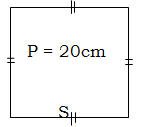4S     =        20cm

4S = 20cm

4                  4

S       =        5cm

Find its area.                                Area   =        Side x Side

=        S x S

=        5cm x 5cm

=        25cm

1. Find the value of x in the figure.S + S + S + S        =        24m

P = 24m      x

x + x + x + x         =        24m

4x      =        24m

4x = 24m

4                 4

X       =        6m

WEEK  NINE.

LESSON  FOUR.

Find the area of the figure.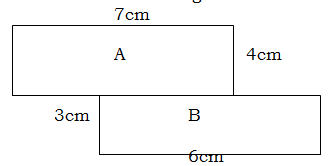Area   =        Area of A     +        Area of B

=        (L  x W)       +        (L x W)

=        (7cm x 4cm)          +        (6cm x 3cm)

=        28cm2 +        18cm2

=        46cm2

Subtraction of area

Given the figure below, use it to answer the questions that follow.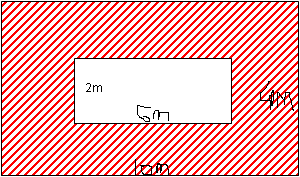1. Find the area of the small rectangle.

A   =        L x W

=        6m x 2m

=        12m2

1. Calculate the area of the big rectangle.

A   =        L x W

=        10m x 4m

=        40m2

1. Find the area of the shaded part.

Area of the shaded part  = Area of big rectangle – Area of small rectangle

=     40m2 –  12m2

4 0

– 1 2

2 8m2

### ASSIGNMENT : Measures assignmentMARKS : 10  DURATION : 1 week, 3 days

SEE ALL
•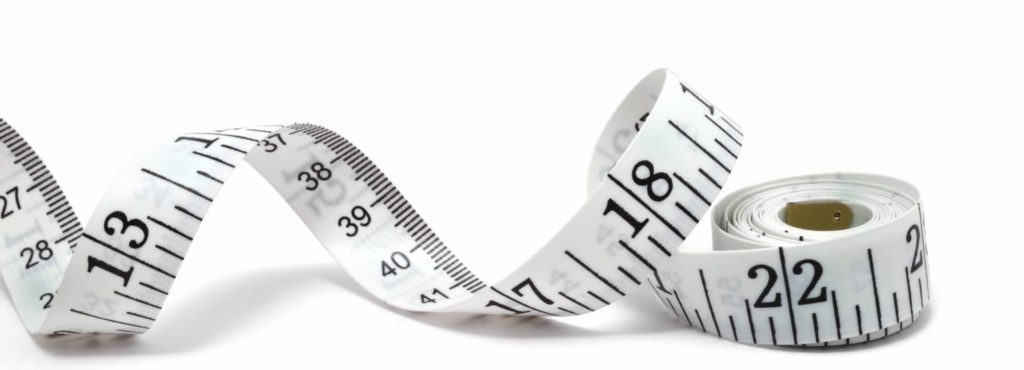YOU